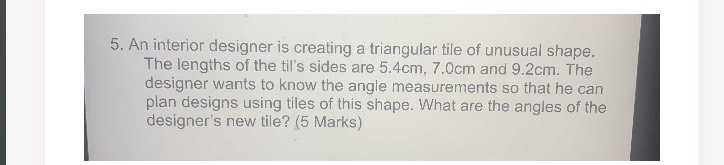### Still have math questions?

Trigonometry
QuestionAn interior designer is creating a triangular tile of unusual shape. The lengths of the til's sides are $$5.4 cm , 7.0 cm$$ and $$9.2 cm$$ . The designer wants to know the angle measurements so that he can plan designs using tiles of this shape. What are the angles of the designer's new tile?

Obtuse Scalene Triangle Side $$a = 7$$ Side $$b = 5.4$$
Side $$c = 9.2$$
Angle $$\angle A = 49.294 ^ { \circ } = 49 ^ { \circ } 17 ^ { \prime } 39 ^ { \prime \prime } = 0.86035 rad$$
Angle $$\angle B = 35.789 ^ { \circ } = 35 ^ { \circ } 47 ^ { \prime } 19 ^ { \prime \prime } = 0.62463 rad$$
Angle $$\angle C = 94.917 ^ { \circ } = 94 ^ { \circ } 55 ^ { \prime } 2 ^ { \prime \prime } = 1.65662 rad$$